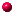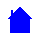## EL10A - Circuit AnalysisLECTURE NOTESunification theories, conservation principleSI measurement - charges and currentintroduction to voltage, energy and powerseries and parallel resistors, Kirchhoff's Lawsvoltage and current sourcescapacitors and inductors in series and parallelNodal AnalysisNodal Analysis ExampleMesh AnalysisSupernode ExampleSuperposition ExampleSolving linear systems - Cramer's ruleSolving linear systems - Gaussian eliminationSolving linear systems - Dividing a vector by a matrixComparison of Node and Mesh methods on hybrid circuitSolving the equations for the hybrid circuitMaximum Power Transfer theoremThevenin and Norton theoremsThe natural response of a first order circuit - CapacitorThe natural response of a first order circuit - InductorResponse of a first order circuit to Constant Forcing Function - CapacitorResponse of a first order circuit to Constant Forcing Function - InductorExample of solving in First Order CircuitsExample illustrating Transient and Steady-State response componentsExample of solving in Second Order CircuitsThe parallel RLC circuitThe series RLC circuitA.C. circuit calculations using trigonometryA.C. circuit calculations using complex numbersthe parallel tank circuit (added on 26 JULY, 2003)Definitions

Contact Stephen Mendes directly at 230-4818 or 266-8027EL10A Homepage